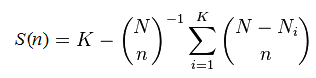Abundance rarefaction

Suppose you observe K different species, finding Nk individuals in the kth species. The sum N = N1+ N2+ ... + NK is the total number of observations. To calculate the rarefaction curve for (number of observed species) vs. (number of observations), we take random subsets with n =1, 2, ... N observations and count the number of species S(n) that are found in each subset. (More accurately, we should average over many different random subsets at each size n until the mean converges). Some species will disappear with fewer observations, and the curve will approach zero species as n approaches zero. It is not actually necessary to implement subsampling and average, S(n) can be calculated using this formula:My colleague Henrik Flyvbjerg and I have developed the modified formula for S(n) when singletons are discarded; let me know if you are interested and I will send you the details.

Rarefaction for OTUs
If species with exactly one observation are ignored, then the above formula does not apply. Thus, if singleton reads are discarded, as recommended in the UPARSE pipeline, then you cannot use standard rarefaction software and the above formula does not give the correct result. If singletons are retained, then the formula is a reasonable approximation, but is not exactly correct because de novo OTUs are necessarily "unstable", meaning that a given pair of sequences may belong to the same OTU with one subset but in different OTUs in another subset.

With de novo OTUs, including those made by UPARSE, then strictly speaking, rarefaction curves must be generated by running the pipeline from scratch for each random subsample and noting the number of OTUs obtained.